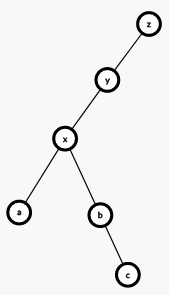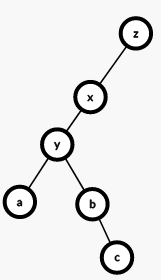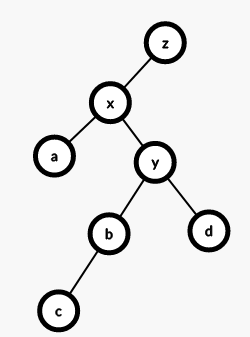# 前言

## 进入正题

(Splay)算法中，一个处处都要用到的东西就是旋转，即将当前节点与其前边一个节点依次旋转到目标位置。由于这个树是一个二叉搜索树，所以旋转之后要保证性质不变。我们就需要找到当前节点的父亲和爷爷节点，然后先更新爷爷节点与当前节点之间的关系，然后将父亲节点与该节点所属关系的另一个子树连起来，最后再处理一下该节点和父亲节点的关系。``void rotate(int x){//旋转 	int y=t[x].fa; 	int z=t[y].fa; 	int k=t[y].ch==x;//找到x是y的左还是右节点，便于进行上文中所说操作 	t[z].ch[t[z].ch==y]=x;//以下都是上边说过的操作。 	t[x].fa=z; 	t[y].ch[k]=t[x].ch[k^1]; 	t[t[x].ch[k^1]].fa=y; 	t[x].ch[k^1]=y; 	t[y].fa=x; 	pushup(y); 	pushup(x); } void splay(int x,int goal){//旋转 	while(t[x].fa!=goal){//父亲节点不是目标 		int y=t[x].fa; 		int z=t[y].fa; 		if(z!=goal){//爷爷节点也不是目标 			(t[y].ch==x)^(t[z].ch==x)?rotate(x):rotate(y);//其中一个点的左儿子是x就翻转x，不然翻转y（因为树有序，不能破坏性质） 		}//上边这句话还需要细细钻研，本题解以后还会完善 		rotate(x); 	} 	if(goal == 0){//到了根节点的话让根节点更新 		root = x; 	} } ``

## 一些操作

``struct Node {        int ch;//子节点        int fa;//父节点         int cnt;//数量        int val;//值         int son;//儿子数量   }t[maxn]; ``

### (1)、插入

``void Add(int x){ 	int u=root,fa=0; 	while(u&&t[u].val!=x){ 		fa=u; 		u=t[u].ch[x>t[u].val]; 	} 	if(u){//有的话直接大小加一 		t[u].cnt++; 	} 	else{//没有该值的节点 		u=++tot; 		if(fa)t[fa].ch[x>t[fa].val]=u; 		t[tot].ch=0; 		t[tot].ch=0; 		t[tot].val=x; 		t[tot].fa=fa; 		t[tot].cnt=1; 		t[tot].son=1; 	} 	splay(u,0); } ``

### (2)、查找值为(x)的排名：

``void Find(int x){ 	int u=root;//从根开始 	if(!u)return;//没有树就直接跳出 	while(t[u].ch[x>t[u].val] && x!=t[u].val){//依次向下找到当前值的点 		u=t[u].ch[x>t[u].val];//更新 	} 	splay(u,0);//旋转 } ``

### (3)、求前驱后继（这个操作求出来的是节点编号）

``int Fr_last(int x,int flag){//前驱flag为0,后继为1 	Find(x);//找到位置 	int u=root;//根开始 	if((t[u].val>x&&flag) || (t[u].val<x && !flag)){//当前点满足就直接返回 		return u; 	} 	u=t[u].ch[flag];//向目标点（左或右）转移 	while(t[u].ch[flag^1])u=t[u].ch[flag^1];//找到转移后最接近当前值的点 	return u;//返回 } ``

### (4)、查找排名为(x)的值

``int Find_thval(int x){ 	int u=root;//根开始 	if(t[u].son<x){//如果没有这么多，直接返回0 		return 0; 	} 	while(666666){//一直循环 		int y=t[u].ch;//记录左儿子 		if(x>t[y].son+t[u].cnt){//排名大就减去，然后走到右节点 			x-=t[y].son+t[u].cnt; 			u=t[u].ch; 		} 		else{ 			if(x<=t[y].son){//否则走到左节点 				u=y; 			} 			else return t[u].val;//如果排名比上边的小，且比左节点的值大，这就是满足的价值，直接返回 		} 	} } ``

### (5)、删除

``void Delete(int x){ 	int Front=Fr_last(x,0);//前驱 	int Last=Fr_last(x,1);//后继 	splay(Front,0);//旋转 	splay(Last,Front); 	int del=t[Last].ch;//找到需要删除的点 	if(t[del].cnt>1){//大于1直接减 		t[del].cnt--; 		splay(del,0); 	} 	else{//否则直接删除 		t[Last].ch=0; 	} } ``

## 总结

### 板子题代码：

``#include<bits/stdc++.h> using namespace std; const int maxn = 5e5+10; const int Inf = 2147483647; struct Node{ 	int son,ch,fa,cnt,val; }t[maxn]; int n,tot,root; void pushup(int x){ 	t[x].son = t[t[x].ch].son+t[t[x].ch].son+t[x].cnt; } void rotate(int x){ 	int y=t[x].fa; 	int z=t[y].fa; 	int k=t[y].ch==x; 	t[z].ch[t[z].ch==y]=x; 	t[x].fa=z; 	t[y].ch[k]=t[x].ch[k^1]; 	t[t[x].ch[k^1]].fa=y; 	t[x].ch[k^1]=y; 	t[y].fa=x; 	pushup(y); 	pushup(x); } void splay(int x,int goal){ 	while(t[x].fa!=goal){ 		int y=t[x].fa; 		int z=t[y].fa; 		if(z!=goal){ 			(t[y].ch==x)^(t[z].ch==x)?rotate(x):rotate(y); 		} 		rotate(x); 	} 	if(goal == 0){ 		root = x; 	} } void Find(int x){ 	int u=root; 	if(!u)return; 	while(t[u].ch[x>t[u].val] && x!=t[u].val){ 		u=t[u].ch[x>t[u].val]; 	} 	splay(u,0); }  void Add(int x){ 	int u=root,fa=0; 	while(u&&t[u].val!=x){ 		fa=u; 		u=t[u].ch[x>t[u].val]; 	} 	if(u){ 		t[u].cnt++; 	} 	else{ 		u=++tot; 		if(fa)t[fa].ch[x>t[fa].val]=u; 		t[tot].ch=0; 		t[tot].ch=0; 		t[tot].val=x; 		t[tot].fa=fa; 		t[tot].cnt=1; 		t[tot].son=1; 	} 	splay(u,0); } int Fr_last(int x,int flag){ 	Find(x); 	int u=root; 	if((t[u].val>x&&flag) || (t[u].val<x && !flag)){ 		return u; 	} 	u=t[u].ch[flag]; 	while(t[u].ch[flag^1])u=t[u].ch[flag^1]; 	return u; } void Delete(int x){ 	int Front=Fr_last(x,0); 	int Last=Fr_last(x,1); 	splay(Front,0); 	splay(Last,Front); 	int del=t[Last].ch; 	if(t[del].cnt>1){ 		t[del].cnt--; 		splay(del,0); 	} 	else{ 		t[Last].ch=0; 	} } int Find_thval(int x){ 	int u=root; 	if(t[u].son<x){ 		return 0; 	} 	while(666666){ 		int y=t[u].ch; 		if(x>t[y].son+t[u].cnt){ 			x-=t[y].son+t[u].cnt; 			u=t[u].ch; 		} 		else{ 			if(x<=t[y].son){ 				u=y; 			} 			else return t[u].val; 		} 	} } int main(){ 	int n; 	Add(Inf); 	Add(-Inf); 	scanf("%d",&n); 	while(n--){ 		int opt; 		scanf("%d",&opt); 		int x; 		if(opt == 1){ 			scanf("%d",&x); 			Add(x); 		} 		if(opt == 2){ 			scanf("%d",&x); 			Delete(x); 		} 		if(opt == 3){ 			scanf("%d",&x); 			Find(x); 			int ans = t[t[root].ch].son; 			printf("%dn",ans); 		} 		if(opt == 4){ 			int ans; 			scanf("%d",&x); 			ans = Find_thval(x+1); 			printf("%dn",ans); 		} 		if(opt == 5){ 			scanf("%d",&x); 			int ans = Fr_last(x,0); 			printf("%dn",t[ans].val); 		} 		if(opt == 6){ 			scanf("%d",&x); 			int ans = Fr_last(x,1); 			printf("%dn",t[ans].val); 		} 	} 	return 0; } ``

Vacanda （HZOIer）资料部分资料来自网络，侵权毕设源码联系删除

qklbishe.com区块链毕设代做网专注|以太坊fabric-计算机|java|毕业设计|代做平台 » Vacanda （HZOIer）资料